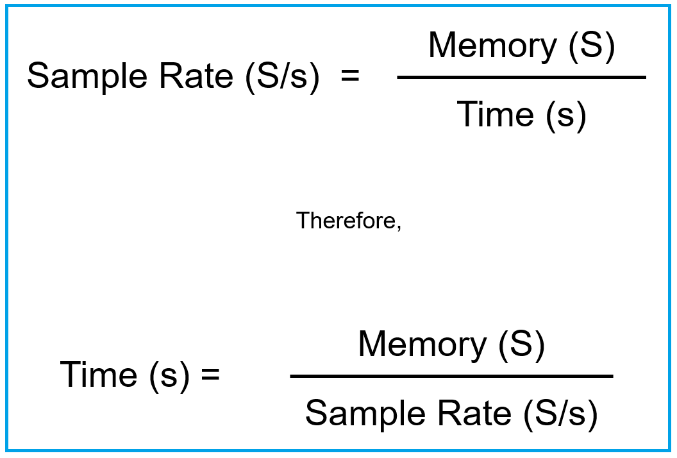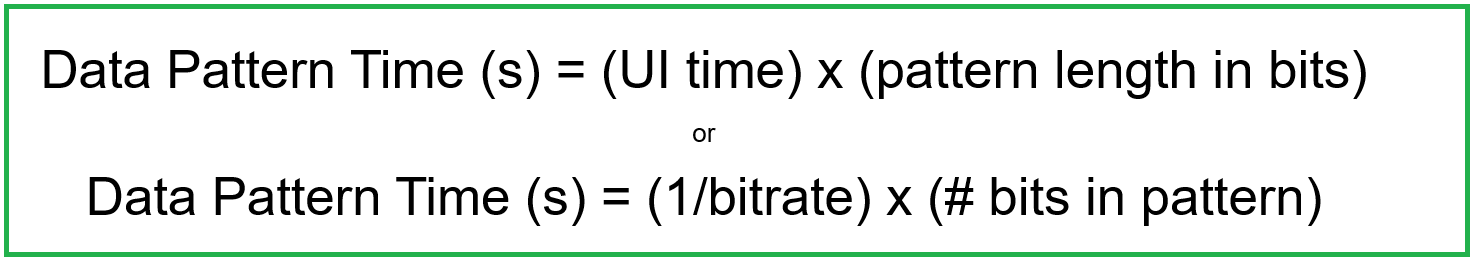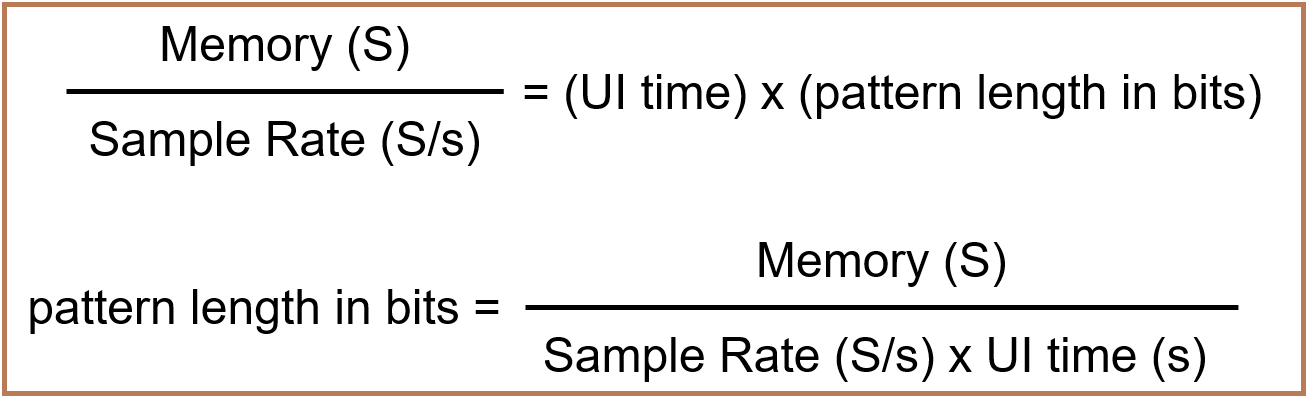Oscilloscopes are increasingly used as a tool for capturing and analyzing high speed serial data transmissions. A natural question arises as to how long of a serial data pattern can be captured using an oscilloscope. The method for determining this maximum serial data pattern length has historically not been well documented and is interdependent on the combination of the following four factors: (1) the specified signal bit rate, (2) the maximum available oscilloscope acquisition memory, (3) the user-selected choice of acquisition sample rate, and (4) the device under test's specified signal data pattern length. This article provides a unified equation and quick rule of thumb for signal integrity engineers to determine how long of a pattern can be captured using their oscilloscope.

### Sampling Rate and Time Capture Window

Oscilloscope hardware has a specified maximum sampling rate and maximum acquisition memory length, but are often operated at a lower, user-specified sampling rate. The choice of sampling rate directly impacts the amount of time that can be captured during one acquisition.

Sampling rate for a given acquisition is the ratio of the amount of memory used for that acquisition divided by the time capture window size. The time capture window size is therefore determined as shown in Figure 1.Figure 1. Equations relating sample rate, memory, and time capture window for an oscilloscope acquisition

For serial data transmissions, the amount of time corresponding to one pattern length is determined by the unit interval time (also referred to as one bit period) multiplied by the pattern length in bits. This is equivalent to the inverse of the bitrate multiplied by the number of bits in the pattern as shown in Figure 2.Figure 2. Calculation for data pattern time

As an example, using the equation in Figure 2, the data pattern time for a 5 Gb/s PRBS15 pattern is (200 ps) x (215) = 200 E-12 x 32768 = 6.5535 us. Using the same equation, a 10 Gb/s PRBS21 pattern time is (100 ps) x (221) = 100 E-12 x 32768 = 209.72 us.

By combining the equations from both Figures 1 and 2, the pattern length in bits can be constrained by the oscilloscope scope memory and sample rate values as shown in Figure 3.Figure 3. Combining equations expresses a relationship between scope memory, scope sample rate, UI time, and pattern length in bits

To solve for the maximum bit sequence (PRBS) pattern that can be acquired using an oscilloscope with 80 GS/s sample rate, 512 Mpts memory, and a signal bitrate of 10 Gb/s, the pattern length in bits that can be captured is (512 Mpts) / (80 Gpts/s x 100 ps/bit) = 64 million bits.

Using these values and solving for the PRBS pattern length, 2N = 64E+6. Solving for N = log2(64E+6), yields N = 25.9. This confirms that the maximum 10 Gb/s PRBS pattern length that can be captured using a sample rate of 80 GS/s, and memory length of 512 Mpts on an oscilloscope is a PRBS25 signal.

Can an oscilloscope capture a PRBS31 pattern? Currently, the longest contiguous oscilloscope memory is 5 Gpts.  Using the equation in Figure 3 and setting the pattern length to 231, the maximum ratio of sampling rate to bitrate is ((5E9) / (231)) = 2.3283 samples per bit. Therefore, although an oscilloscope can capture a full PRBS31 pattern length, with just over 2.3 samples per bit, the signal would be undersampled.

In summary, the unified equations in this article relating signal bitrate, signal pattern length, scope sampling rate, scope memory, and event capture time can be used by signal integrity engineers to determine the answer to many questions, including how many repetitions of a pattern can be captured, how long of a pattern can be captured, and what sample rate and memory length are needed given the target event capture window size.